# Generate Random Character Strings

This post will guide you how to generate a random character string in excel. How do I create a random passwords, text strings with a formula in excel. How do I generate random strings of characters with VBA code in excel. How to generate random alphanumeric values in excel.

## Generate Random Character Strings with Formula

To generate random character strings in excel, you should be generate random numbers, letters, and special characters. We have talked that how to generate a random number string with Excel RANDBETWEEN function in excel.

Generate random Number

To generate random number string, you can use a formula based on the RANDBETWEEN function. like this:

`=RANDBETWEEN(0,9)`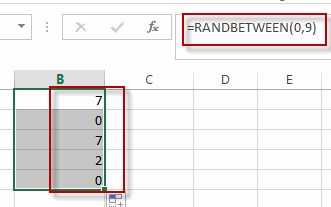This function will generate a random number between 0 and 9.

Generate random Letter String

To generate random uppercase or lowercase letter string in excel, you can create a formula base on the CHAR function and the RANDBETWEEN function. like this:

`=CHAR(RANDBETWEEN(65,90))`

or

`=CHAR(RANDBETWEEN(97,122))`

The first formula will generate random uppercase letter between A and Z.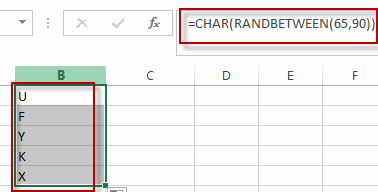The second formula will generate random lowercase letter between a and z.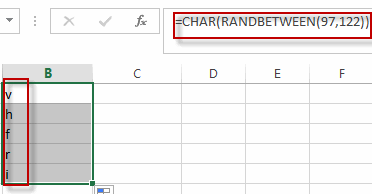Generate Random Specific Character

To generate random specific character in excel, you can use the below formula:

`=CHAR(RANDBETWEEN(33,47))`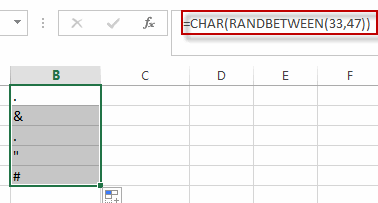This formula will generate random specific characters, such as: #\$!/

Generate random 5-digit number

To generate a random 5-digit number,you can use the following formula:

`=RANDBETWEEN(10000,99999)`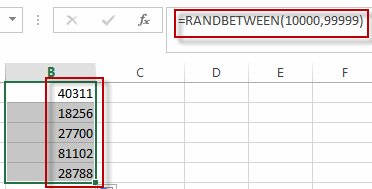Generate Random Three Letters String

We have know how to create a random uppercase letter and lowercase letter, if you want to create a random 3-letters string, you can use the following formula:

`=CHAR(RANDBETWEEN(65,90))& CHAR(RANDBETWEEN(65,90)) & CHAR(RANDBETWEEN(65,90))`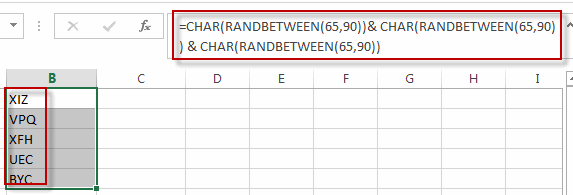## Generate Random Character String with VBA Code

You can also use an VBA Macro Code to generate a random character string in a selected cell in Excel. You just need to do the following steps:

#1 open your excel workbook and then click on “Visual Basic” command under DEVELOPER Tab, or just press “ALT+F11” shortcut.#2 then the “Visual Basic Editor” window will appear.

#3 click “Insert” ->”Module” to create a new module#4 paste the below VBA code into the code window. Then clicking “Save” button.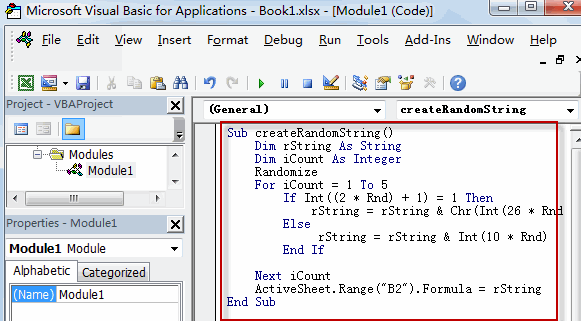```Sub createRandomString()
Dim rString As String
Dim iCount As Integer
Randomize
For iCount = 1 To 5
If Int((2 * Rnd) + 1) = 1 Then
rString = rString & Chr(Int(26 * Rnd + 65))
Else
rString = rString & Int(10 * Rnd)
End If

Next iCount
ActiveSheet.Range("B2").Formula = rString
End Sub```

#5 back to the current worksheet, then run the above excel macro. Click Run button.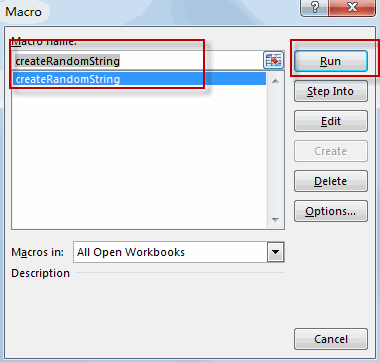#6 Let’s see the result.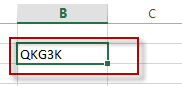### Related Functions

• Excel CHAR function
The Excel CHAR function returns the character specified by a number (ASCII Value).The CHAR function is a build-in function in Microsoft Excel and it is categorized as a Text Function. The syntax of the CHAR function is as below:=CHAR(number)….
• Excel RANDBETWEEN Function
The Excel RANDBETWEEN function returns a random integer number that is between the numbers you specify.The syntax of the RANDBETWEEN function is as below:= RANDBETWEEN (bottom,top)….